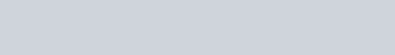### Prefix Code

It is a type of code system distinguished by its possession of the “prefix property”, which requires that there is no whole code word in the system that is a prefix (initial segment) of any other code word in the system. For example, a code with code words {9, 55} has the prefix property; a code consisting of {9, 5, 59, 55} does not, because “5” is a prefix of “59” and also of “55”. A prefix code is a uniquely decodable code: given a complete and accurate sequence, a receiver can identify each word without requiring a special marker between words.

### Huffman coding

It is a lossless data compression algorithm which is based on Prefix code, where variable-length codes is assigned to input characters such a way that the code assigned to one character is not prefix of code assigned to any other character.

The structure of the tree can be used to determine the coding of any leaf by using the 0/1 edge convention. If we use a different tree, we get a different coding. As an example, in the below tree all characters are stored at the leaves of a complete tree.Encoding of th tree is

charbinary
‘g’10
‘o’11
‘p’0100
‘h’0101
‘e’0110
‘r’0111
‘s’000
‘  ‘001

Using this coding, “go go gophers” is encoded (spaces wouldn’t appear in the bitstream) as:
10 11 001 10 11 001 10 11 0100 0101 0110 0111 000

### Example

The character-encoding induced by the tree can be used to decode a stream of bits as well as encode a string into a stream of bits. To decode the below stream, start at the root of the encoding tree, and follow a left-branch for a 0, a right branch for a 1. When you reach a leaf, write the character stored at the leaf, and start again at the top of the tree. Continuing until all the bits are processed yields

Stream : 01010110011100100001000101011001110110001101101100000010101 011001110110
Encoding : her sphere goes here

### Huffman’s algorithm

Each character has an associated weight equal to the number of times the character occurs, for example. In the “go go gophers” example, the characters ‘g’ and ‘o’ have weight 3, the space has weight 2, and the other characters have weight 1. Huffman’s algorithm assumes that we’re building a single tree from a group (or forest) of trees. Initially, all the trees have a single node with a character and the character’s weight. Trees are combined by picking two trees, and making a new tree from the two trees. This decreases the number of trees by one at each step since two trees are combined into one tree. The algorithm is as follows:

1. Begin with a forest of trees. All trees are one node, with the weight of the tree equal to the weight of the character in the node. Characters that occur most frequently have the highest weights. Characters that occur least frequently have the smallest weights.
2. Repeat this step until there is only one tree:
3. Choose two trees with the smallest weights, call these trees T1 and T2. Create a new tree whose root has a weight equal to the sum of the weights T1 + T2 and whose left subtree is T1 and whose right subtree is T2.
The single tree left after the previous step is an optimal encoding tree.

We’ll use the string “go go gophers” as an example. Initially we have the forest shown below. The nodes are shown with a weight/count that represents the number of times the node’s character occurs.Pick two minimal nodes. There are five nodes with the minimal weight of one, it doesn’t matter which two we pick. We create a new tree whose root is weighted by the sum of the weights chosen. We now have a forest of seven trees as shown here:Choosing two minimal trees yields another tree with weight two as shown below. There are now six trees in the forest of trees that will eventually build an encoding tree.Finally final tree whose weight is thirteen, the sum of the two weights six and seven is shown belowHuffman’s algorithm is an example of a Greedy algorithm. It’s called greedy because the two smallest nodes are chosen at each step, and this local decision results in a globally optimal encoding tree. In general, greedy algorithms use small-grained, or local minimal/maximal choices to result in a global minimum/maximum.